## Applying to Grad School: Slow and Steady (and Early) Wins the RaceThis could be you!

The early bird always gets his worm.  Early to bed and early to rise, makes a man healthy, wealthy, and wise.  I think by now you get the picture.  As fully developed adults with fully established adult habits and careers (what’s the term everyone’s using these days? Adulting?), we have by now learned that procrastination can be a formidable foe.  My sincerest apologies for the deluge of age-old idioms and rinse-and-repeat motivational quotes, but let’s be crystal clear: you need to begin the process ASAP.  How soon?  If you are applying to fall admission for Graduate Studies, you need to begin the process in the spring semester of the previous year.  For those of us who are not so future-oriented: if you are applying to Graduate School for the fall 2019 Semester, you need to begin your application process in the spring of 2018.  Today is February 2, 2018, so that means like, NOW!

Just like you, I am currently beginning the Graduate School application process, which, I have come to realize, is one that requires a series of well-planned out steps.  But where do you begin? Continue reading “Applying to Grad School: Slow and Steady (and Early) Wins the Race” »

The test gurus at Testmasters are at it again with more tricks and tips for conquering the GRE. Today’s problem involves questions with multiple answers. Both paper exams and the computerized test will have math questions that could have more than one correct answer, and to get credit for answering the question correctly, you will have to give all of the answers for that question. Many of these problems are algebra based, such as the one below.

If the numbers 31, 24, and x are averaged, the value will be somewhere between 20 and 30, inclusive. Which of the values below could be x?

1. 4
2. 5
3. 20
4. 35
5. 40

To solve this problem as quickly as possible, without plugging in all five answers, find the smallest and largest possible values for x based on the largest and smallest possible averages for the set of numbers. Recall that an average is the sum of terms divided by the number of terms. Plug in the information from the problem into this, so the average equals the lowest value of 20.Solve for x by first multiplying both sides by 3.The lowest possible value for x is 5, which eliminates answer (A) as a possible choice, and it verifies that (B) is one of the correct answers.

Now, repeat the process with the largest value for the average, 30.Solve for x by first multiplying both sides by 3.This means that the largest value for x is 35, so any answer choices between 5 and 35, inclusive will be answers. For this problem, you would select answers (B), (C), and (D).

## Sample GRE Multiple Choice Math Problem – Mean Means

On every GRE Math section, the test makers try to come up with a few extremely difficult problems that will leave even the cleverest students scratching their heads. The really evil part, though, is that even these problems can be solved in under a minute without a calculator – if you know what to do. This means that once you “figure out the trick,” these difficult problems become easy. So, while those test makers are busy cackling with sadistic glee, let’s see if we can’t beat them at their own game.

Consider the following problem:

If the average (arithmetic mean) of four different positive integers is 5, then the least possible product of the four integers is:

A) 20

B) 84

C) 104

D) 480

E) 504

This one might seem like a hard nut to crack. After all, there are many possible sets of four distinct positive integers that have an average of 5. We could not possibly test them all one by one given the time constraints of the GRE. So how are we going to figure out which four integers are the ones that yield the smallest possible product?

Well, if we want the smallest product, then we want as many of the integers to be as small as possible. If the smallest of the three integers were equal to 1, then that would be great, since multiplying by one simply produces the same number and would thus not increase the product of the three numbers. Since the four integers must be distinct, we can make the second integer 2 so that it is also as small as possible, and by the same logic the third integer would be 3. All that remains is to determine the fourth integer, which we can find using algebra:The fourth integer is 14, and the product is:Logically, the answer must be 84, or choice B. If you are still feeling unsure, you could try a few other sets of four integers to see if their products are less than 84. What happens, for instance, when we increase the 3 to 4 and decrease the 14 to 13?Already, the product begins to increase. Try any other set and you will see that its product is greater than 84. It doesn’t matter that we decreased 14 to 13, because what controls the outcome in this problem is how small we can make the smallest numbers in the set. Thus, our original reasoning was correct.

If you know what to do, it takes only about 30 seconds to solve this problem. So you see, with practice, even the hardest problems on the GRE become easy. Check back here each week for more extra hard problems and the tricks you need to solve them! Also, remember that you can find out all the tricks from experts like me with a Test Masters course or private tutoring. Until  then, keep up the good work and happy studying!

## Quantitative Reasoning: Making Ratios Rational

The GRE quantitative reasoning section contains many different types of questions, some of which can be on the difficult side, but the GRE prep experts at Testmasters are here to help you through the ins and outs of solving even the hardest of math problems on this exam.

Today, we look at ratios. Though there are many forms, most ratios are given in either fraction form or with a colon, but both forms are comparing parts to parts. The secret to most ratio problems is to first identify the total number of parts that you’re dealing with. Once that is known, you can take those parts and use the total to find fractional amounts or to solve for probability.

Here is an example of how the total in a ratio problem can help you to find a fractional amount:

A cocktail contains various ingredients: club soda, hard apple cider, brandy, and vodka. A bartender uses a ratio of 9:5:4:2 respectively to mix the ingredients by volume. How much vodka should the bartender use to prepare a 6-oz drink?

Solve this problem by first finding the total number of parts. Simply add the ratio amounts together to get the total:

9+5+4+2=20

Recall that a fraction is equal to the part over the total. The part of the mixture that is vodka is 2, and the total number of parts are 20; therefore, the fraction of the drink that is vodka is.

Multiply this fraction by the total number of ounces in the final drink to determine the amount the bartender should pour into a 6-oz drink.There will only be 0.6 oz. of vodka in a 6-oz. drink, and using this same method, you could solve for the amounts of the other ingredients, too. Try it yourself. How many ounces of club soda, hard apple cider, and brandy would each be in the 6-oz. drink? The answers are below. No peeking until after you’re done.

Club soda:  2.7 ouncesHard apple cider: 1.5 ouncesBrandy: 1.2 ounces## Sample GRE Multiple Choice Math Problem – Lost in Translation

On every GRE Math section, the test makers try to come up with a few extremely difficult problems that will leave even the cleverest students scratching their heads. The really evil part, though, is that even these problems can be solved in under a minute without a calculator – if you know what to do. This means that once you “figure out the trick,” these difficult problems become easy. So, while those test makers are busy cackling with sadistic glee, let’s see if we can’t beat them at their own game.

Consider the following problem:

The average of four numbers is five less than the average of the three numbers that remain after one has been eliminated. If the eliminated number is 2, what is the average of the four numbers?

A) 17

B) 22

C) 66

D) 68

E) 100

“What numbers?” you ask, “How am I supposed to find the averages of numbers that no one knows?!”

The GRE test makers love to make math questions harder by forcing you to work with variables instead of with actual numbers. Actual numbers are concrete, intuitive, and easy to understand, while variables are more abstract and difficult to grasp. At least that’s their theory. As you’ll see, variables aren’t actually harder than actual numbers. In fact, in some cases, problems that have many unknowns can actually be faster and easier to solve than problems with actual numbers, since there is less to figure out (because there’s less you can figure out). The main trick comes at the beginning, when we have to translate the words of a problem into a mathematical expression we can manipulate on our scratch paper.

First, the problem tells us that we have “the average of four numbers.” Well, how do you find the average of four numbers? You add them up and divide by 4. We can write this down if we pick variables to represent each of the four numbers. Let’s just go with a, b, c, and d.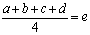Here, e represents the average of the four numbers (what we are trying to find). Next, the problem tells us that we take the average of 3 of the four numbers. This mean we have to leave out one of our variables. Since d is the last one, we’ll leave it out: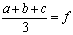The new average is represented by f. Now the problem also tells us that the variable that was left out was equal to 2, so we know that: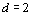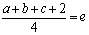The problem also tells us that the average of four numbers is five less than the average of the three numbers that remain after one has been eliminated. We have let e represent the average of the four numbers and f represent the average of the three numbers, so we could rephrase this as e is five less than f: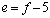So, now we still have all of these variables. We want to substitute variables for each other until we have one equation with one variable, because then we can solve for that variable and hopefully get closer to solving for e. Here’s one possible way to do that: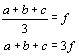We can now substitute 3f for a+b+c in the other equation: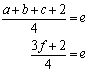We can also substitute f – 5 for e, since we know that e = f – 5: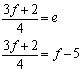Now we have one equation with only one variable, so we can solve for f: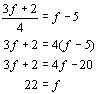Of course, we are trying to solve for e, so we should plug this into the equation e = f – 5: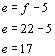Thus, the correct answer is choice A. Note, however, that if you had only solved for f, 22 was a choice as well. With this problem, it’s all about translating the words into variables and then moving the variables around until you can actually solve for one of them. This problem might be a little more time consuming than some of the others we’ve looked at in this series, but it can still be solved in under a minute without a calculator, if you know what to do. So you see, with patience and practice, even the hardest problems on the GRE become easy. As you do more practice problems you will get better and better at them – the test makers tend to use the same tricks over and over again. Check back here each week for more extra hard problems and the tricks you need to solve them! Also, remember that you can find out all the tricks from experts like me with a Test Masters course or private tutoring. Until  then, keep up the good work and happy studying!

## Quantitative Comparison: Secrets of Surface Area and Volume in a Comparison Problem

It’s time to once again practice a problem for the GRE exam. This time, the professional GRE preppers at Testmasters bring you a step-by-step solution to solving surface area, volume, and quantitative comparison problems.

First, a brief note about quantitative comparison problems. For these problems, the questions can come from any area of math, but you will have to determine which quantity is larger, if both are equal, or if a relationship cannot be determined with the given information. Sometimes, you will have to calculate values for each quantity to compare them, but other times, you will not be able to find exact values because there may be variables involved. In these instances, it helps to know what happens when certain math operations are performed.

Today’s problem is a quantitative comparison problem that uses surface area and volume of a cube. The GRE does not provide any formulas, so you will have to know them before taking the exam. If you write the formulas down every time you use them, memorizing them becomes much easier.

Below are two quantities. If quantity A is larger, the answer is A. If quantity B is larger, the answer is B. If they are both equal, the answer is C, and if a relationship cannot be determined, the answer is D.

The length of a side of cube A measures 4, and the length of each side of cube B measures 3.

Quantity A

The ratio of the surface area of cube A to the volume of cube A.

Quantity B

The ratio of the surface area of cube B to the volume of cube B.

First, find the surface area of each of the cubes. The surface area is equal to. Plug in the side length for each of the cubes into the formula.Now, recall that the volume of a cube is. Plug in the side lengths for each of the cubes into this volume formula to find the volumes of the respective cubes.Because the question asked for the ratio of each cube’s surface area to its volume, divide the surface area of cube A by its volume and repeat the process for cube B.Because the ratio of the surface area of cube B to its volume is greater than the same ratio for the surface area and the volume of cube A, the answer is (B).

## GRE Vocab and the Seven Deadly Sins: Part VII – PrideAn early sixteenth century Dutch depiction of the seven deadly sins, by a follower of Heironymus Bosch.

Finally, we come to the end of our series with the last and deadliest of the seven deadly sins: pride. While in English the word “pride” can often have a positive connotation, as in “the parents are proud of their child,” this kind of pride is something else altogether. In Latin, this sin is referred to as superbia, which perhaps gives a clearer indication of its nature than does the English equivalent. Pride is defined by the Catholic church as the belief that one is innately superior to others, especially in the sense that the sinner feels that he or she does not have to act with regard to the well-being of others because he or she is “better” than they are. Pride is thus a sin of selfishness and arrogance that leads one to feel that one is above the rules and that other people don’t matter. You begin to see why this is the worst one. According to Catholic theology, pride is the worst sin of all because it is the source of the other sins. It was, after all, pride that caused the angel Lucifer to rebel against God and become Satan, the Devil himself.

There are many excellent potential GRE vocab words that have to do with the sin of pride, including superbity, vainglory, hubris, haughtiness, hauteur, superciliousness, amour-propre, conceitedness, narcissism, and condescension. Continue reading “GRE Vocab and the Seven Deadly Sins: Part VII – Pride” »

## Quantitative Reasoning Sample: Making Percents Less Perplexing

For the GRE’s quantitative reasoning section, you will have to show your ability to work with both fractions and percents. Occasionally, problems will combine both, but because percents and fractions are closely related, even problems that use both can be solved. The GRE guides at Testmasters bring you today a walkthrough of a common type of percent and fraction problem you could see on the exam.

In an undergraduate program at a university, there were 300 graduates one year. By the time the class reunion rolled around 10 years after graduation, of those 300 students,went on to get their masters degrees, and of those who earned a masters, another 25% continued onto higher education and got their doctorate degrees. Of the 300 original graduates from the undergraduate university program, how many had a doctorate degree at the reunion?

First, break this problem down into its components. Any time you see the word “of” in a fraction or percent problem, it indicates multiplication. Start with the initial amount of graduates and find the number who got their masters. Because the problem indicates thatof the original 300 got a masters degree, turn this into a math equation by replacing the word “of” with a multiplication sign. This makes sense, because in a fraction problem, fraction times the whole is equal to the part.This means that at the reunion, 100 had at least a masters degree.

Next, the problem says that of those who got a masters degree, 25% went on to get a doctorate. Rather than using 300 for the whole, the whole group in this part of the problem is the 100 who got a masters degree. With percent problems, the same formula used for fraction problems applies.At the 10-year reunion, 25 of the original graduates will have a doctorate.

Now, you can conquer percent and fraction problems by remembering the equations: